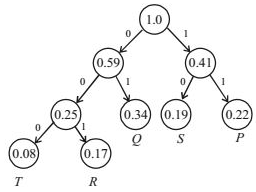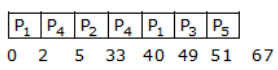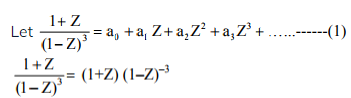# GATE Solved Paper 2017-19 - GATE 2017 Shift 2

>>>>>>>>GATE 2017 Shift 2

 Character Probability P 0.22 Q 0.34 R 0.17 S 0.19 T 0.08 Total 1.00
If a message of 100 characters over X is encoded using Huffman coding, then the expected length of the encoded message in bits is_____.

Note – Numerical Type question

• A

225• B

226• C

227• D

228• Option : A
• Explanation :
By using haffman tree :So, number of bit required for each alphabet:
T = 3 bit, R = 3 bit, Q = 2 bit, S = 2 bit, P = 2 bit
Then, average length per character is = (number of bits * frequency of occurance of each alphabets)
= 3 * 0.08 + 3 * 0.17 + 2 * 0.34 + 2 * 0.19 + 2 * 0.22 = 2.25 bits
And, average length for 100 character = 2.25 * 100 = 225 bits.
Hence, 225 bits is correct answer

 Q1 Q0 Q1+ Q0+ 0 0 0 1 0 1 1 0 1 0 1 1 1 1 1 1
The counter is built as a synchronous sequential circuit using T flip-flops. The expression for T1 and T0 are

• A

T1 = Q1Q0,   T0 = Q1Q0,• B

T1 = QQ0,   T0 = Q1 + Q0,• C

T1 = Q1 + Q0,  T0 = Q1 + Q0,• D

T1 = Q1Q0,   T0 = Q1 + Q0,• Option : B
• Explanation :  Q1 Q0 Q1+ Q0+ T1 T0 0 0 0 1 0 1 0 1 1 0 1 1 1 0 1 1 0 1 1 1 1 1 0 0

 Process Arrival Time Burst Time Priority P1 0 11 2 P2 5 28 0 P3 12 2 3 P4 2 10 1 P5 9 16 4
The average waiting time (in milliseconds) of all the processes using preemptive priority scheduling algorithm is ____.

Note – Numerical Type question

• A

29• B

28• C

30• D

27• Option : A
• Explanation :  Process AT BT Priority P1 0 2 P2 5 0 P3 12 3 P4 2 1 P5 9 4w.t. = 0 + 0 + ( 33 – 5) + (40 – 2) + (49 – 12) + (51 – 9) = 145
Avg wt = 145/5 = 29

• A

Nβ(1 - β)• B• C

N(1 - β)• D

Not expressible in terms of N and β alone• Option : B
• Explanation :
Derivative of gx(z) evaluated at z=1 gives expectation E(X) of X.
Therefore, take derivative of gY(z) with respect to z, and plug in z=1
Derivative is N.β.(1 - β + βz)(N-1), plug in z=1, gives Nβ.

• A

15• B

16• C

16• D

18• Option : A
• Explanation := (1+Z) (1+3Z + 6Z2 + 10Z3 +……….∞)
Using binomial theorem
= 1 + 4Z + 9Z2 + 16Z3 + …… ∞………(2)
From (1) and (2), a0 = 1 and a3 = 16
∴ a3 – a0 = 15
Related Quiz.
GATE 2017 Shift 2